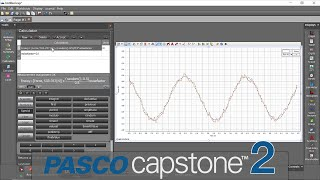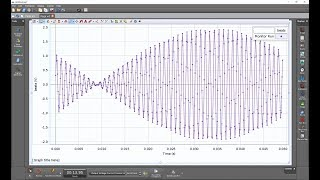# Calculation of Derivative Pt 1--Formulas for Calculation (Capstone)

How does PASCO Capstone calculate the velocity from the position data? PASCO Capstone by default uses a 5 point algorithm to determine the derivative of the position vs time data to determine the velocity. The same type of process is used to determine the acceleration from the calculated velocity data. A full description of the method that is used to calculate the velocity data can be found at the link below.
www.pasco.com/support/technical-support/technote/techIDlookup.cfm?TechNoteID=1221
This algorithm was first implemented within:
Capstone version 1.11.1
SPARKvue version 3.1
MatchGraph 2.1.0

### More "How Do I?" Videos